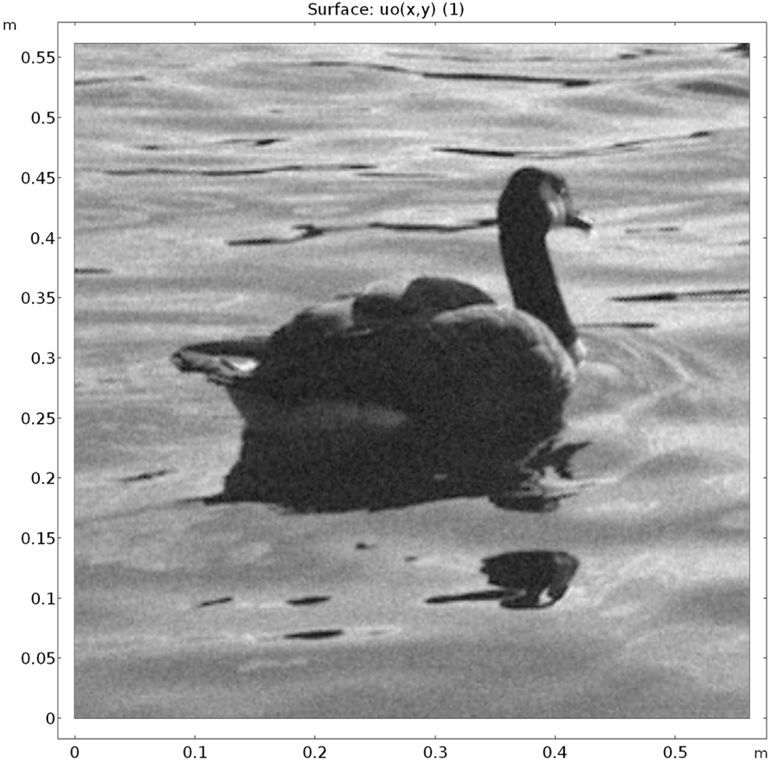# 图像去噪以及其他多维变分问题

2018年 9月 21日

### 高维空间中的变分问题

（之前的讨论中）考虑的是一维问题，并寻找一个函数 u_(x) 使函数最小化

E[u(x)] = \int_a^b F(x,u,u^{\prime})dx.

E[u(x,y,z)] = \int_{\Omega}F(x,y,z,u,u_x,u_y,u_z)d\Omega,

E[u+\epsilon\hat{u}] = \int_{\Omega}
F\left(x,y,u+\epsilon\hat{u},\frac{\partial}{\partial x}(u+\epsilon\hat{u}
),\frac{\partial }{\partial y}(u+\epsilon\hat{u}),\frac{partial } {\partial z}(u+\epsilon\hat{u})\right)d\Omega.

\frac{d}{d\epsilon}\bigg|_{\epsilon=0}E[u+\epsilon \hat u] = 0,

\int_{\Omega} \left[\frac{\partial F}{\partial u}\hat{u}
+\frac{\partial F}{\partial u_x}\hat{u}_x+\frac{partial F} {\partial u_y}\hat{u}_y+\frac{\partial F}{\partial u_z}\hat{u}_z\right]d\Omega = 0, \quad \forall \quad \hat{u}(x,y,z).

\delta E = \int_{\Omega} \delta Fd\Omega=\int_{\Omega} \left[\frac{\partial F}{\partial u}\delta u + \frac{\partial F}{\partial u_x}\delta u_x + \frac{\partial F}{\partial u_y}\delta u_y + \frac{\partial F}{\partial u_z}\delta u_z\right]d\Omega

### 图像去噪：一个多维变分问题

TV(u) = \int_{\Omega}|\nabla u|^2d\Omega = \int_{\Omega}\nabla u \cdot \nabla ud\Omega.

f(u)=\int_{\Omega}(u-u_o)^2d\Omega.

E(u) = \int_{\Omega}\left[ \nabla u \cdot \nabla u + \mu (u-u_o)^2\right]d\Omega

\delta E = \int_{\Omega}\left[2 \nabla u \cdot \delta (\nabla u) + 2\mu (u-u_o)\delta u\right]d\Omega = 0 , \quad \forall \quad \delta u.\int_{\Omega}\left[u_x\hat{u}_x + u_y\hat{u}_y + \mu (u-u_o)\hat{u}\right]d\Omega = 0 , \quad \forall \quad \hat{u}.Tikhonov 正则化会过于平滑，并且不会保留诸如边和角之类的几何特征。由下式给出的 ROF 模型的函数

E(u) = \int_{\Omega}\left[ |\nabla u| + \mu (u-u_o)^2\right]d\Omega

\delta E = \int_{\Omega}\left[ \frac{\nabla u}{|\nabla u|}\cdot \delta (\nabla u) + 2\mu (u-u_o)\delta u\right]d\Omega = 0 \quad \forall \delta u.

ROF 模型保留了边缘，但据说会引起所谓的“阶梯效应”。在函数中包含高阶导数有助于避免这种情况。

### 高阶导数的变分问题

\int\left[\frac{1}{2}EI(\frac{d^2u}{dx^2})^2-fu\right]dx.

\delta \int\left[\frac{1}{2}EI(\frac{d^2u}{dx^2})^2-fu\right]dx = \int\left[EI\frac{d^2u}{dx^2}\delta(\frac{d^2u}{dx^2})-f\delta u\right]dx = 0 \quad \forall \delta u .

### 多未知数变分问题

E(u,v)=\int_{\Omega}F(x,y,z,u,v,u_x,u_y,u_z,\dotsc)d\Omega.

\delta E=\int_{\Omega}\left[\frac{partial F} {\partial u}
\delta u+\frac{\partial F}{\partial v}\delta v + \frac{partial F} {\partial u_x}\delta u_x+\frac{\partial F}{\partial u_y}\delta u_y+\frac{partial F} {\partial u_z}\delta u_z + \frac{\partial F}{\partial v_x}\delta v_x+\frac{partial F} {\partial v_y}\delta v_y+\frac{\partial F} {\partial v_z}\delta v_z+…\right]d\Omega.

E(u,v)=\int_{\Omega}F(x,y,z,u,v,u_x,u_y,u_z,\dotsc)d\Omega.

\mathcal{L}(u,v,\lambda) = \int_{\Omega}\left[F+\lambda g\right]d\Omega,

### 结束语

• 关于变分演算的经典文献：
• I.M. Gelfand and S.V. Fomin (English translation by R.A. Silverman), Calculus of Variations, Dover Publications, Inc., 1963.
• 最近讨论工程问题的文章：
• K.W. Cassel, Variational Methods with Applications in Science and Engineering, Cambridge University Press, 2013.
• J.W. Brewer, Engineering Analysis in Applied Mechanics, Taylor & Francis, 2002.
• 强制约束的策略：
• D.G. Luenberger, Introduction to Linear and Nonlinear Programming, Addison-Wesley Publishing Company, 1973.
• S.S. Rao, Engineering Optimization: Theory and Practice, John Wiley & Sons Inc., 2009.